Hello Guest it is January 23, 2022, 11:10:09 AM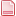Author Topic: Variables reading and writing  (Read 13038 times)

0 Members and 1 Guest are viewing this topic.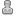Ya-Nvr-No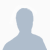•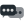992« on: May 17, 2014, 12:05:25 PM »
Been mucking around looking in to reading and writing to variables and trying to figure out the variable#'s
Reality, I was trying to find a way to set the persistent dro's so when I restart it has the same dro coords as when it shut down.
not sure where it picked these from but can not seem to reset them, as the data is held in the xml file not the machine.ini

There has to be a way to write them to memory on shutdown or maybe write them to a file and then read the file and fill the variables with the stored locations.

-- inst 0 is the hobby version 0 is the only one, the pro versions will have more capabilities and Mach4 can then run multiplies instances.
-- this was placed under a button click to read and set, step thru and experiment.
inst= 0

local valx = mc.mcCntlGetPoundVar(inst, 5021);
local valy = mc.mcCntlGetPoundVar(inst, 5022);
local valz = mc.mcCntlGetPoundVar(inst, 5023);
local vala = mc.mcCntlGetPoundVar(inst, 5024);

--how to set variables (in this case to zero)
mc.mcCntlSetPoundVar(inst, 5021, 0);
mc.mcCntlSetPoundVar(inst, 5022, 0);
mc.mcCntlSetPoundVar(inst, 5023, 0);
mc.mcCntlSetPoundVar(inst, 5024, 0);

-- this is a listing of a lot of the variables I found. As you can see there is a pattern to some like kinds
not all as most of course are zero's and there are a lot of them. Your numbers will vary for most.
I labeled some I could get a handle on. Others will have to wait for Documentation.
Writing a while loop to read all the variables > 0 and then writing to a text file or even a wizard is in the plans.

2132=900
2134=10

3005 =200
3101 =3.141592
3102 =2.718282
4001=10
4002 =170
4003 =900
4004 =911
4005 =940
4006 =200
4007 =400
4008 =490
4009 =500
4010 =990
4011 =150
4012 =1
4013 =610

--my machine always defaults to these #' on startup
5021=0.254 --machine coordinate x
5022=1.27 --mc y
5023=1.27 --mc z
5024=50.8 --mc a

5025=453.571429 --not sure what this number is

5111= hs y
5112= hs z
5113= hs a
5114= hs b
5115= hs c

5201= workshift x
5202= ws y
5203= ws z
5204= ws a
5205= ws b
5206= ws c

5221= g54x
5222= g54y
5223= g54z
5224= g54a
5225= g54b
5226= g54c

5241= g55
5261= g56
5281= g57
5301= g58
5321= g59
--and on and on they go as blocks of 20

7785=tool 1 Length
7786=Length wear
7788=1/2 Diameter
7789=1/2 Dia wear

7795=tool 2
7805=tool 3
7815=tool 4
7825=tool 5
7835=tool 6
7845=tool 7
7855=tool 8
7865=tool 9
7875=tool 10
8375=tool 60
8775=tool 100
8785=tool 101
9775=tool 200
9785=tool 201
9795=tool 202
-- and these go on in blocks of 10

10275=tool 250 Length
10276=length Wear
10278=1/2 Dia
10279=1/2 Dia wear

10315=tool 254 Length
10316=length Wear
10318=1/2 Dia
10319=1/2 Dia wear

15000=2418317446859018500000000000000000000000000000000000000000000000000000000000000
15001=2418347089633870200000000000000000000000000000000000000000000000000000000000000
15002=2418376732408721800000000000000000000000000000000000000000000000000000000000000
--up to variable 15038 with those in variations

below are some screen shots of where to look at your variables
and yes you can have multiple ranges and multiple display groups displayed
Pin it to keep them on the top of screen.

--just a random lot of variables and the values
15043=92
15045=17
15046=90
15047=91
15048=94
15049=20
15050=40
15051=49
15052=50
15053=98
15054=15
15055=54
15056=64
15057=97
15058=69
15059=-1.#QNAN0
15060=-1.#QNAN0
15061=-1.#QNAN0
15063=13
15064=10
15065=900
15067=-1
15068=-1
15069=-1
15102=-1
15104=-1
15105=-1.#QNAN0
15106=-1.#QNAN0
15107=-1.#QNAN0
15108=-1.#QNAN0
15109=-1.#QNAN0
15110=-1.#QNAN0
15111=-1.#QNAN0
16127=5000
16288=3600
16293=5

« Last Edit: May 17, 2014, 12:17:04 PM by Ya-Nvr-No »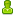BR549•6,952« Reply #1 on: May 17, 2014, 12:22:23 PM »
HIYA Craig, I have a #VAR report generator for MACH3 that does that trick. It sweeps through ALL the Var Numbers and reports ONLY the one that are > OR <  0

IT IS important that you check for values < AND > because some values are negative values.

Save the text file and send it to Notepad for viewing and printing.

I trick I use a lot when trying to find something in the VARs . I input a Value into a DRO that is easy to spot then I create the report and open in Notepad. THen do a search on that string to find the value and I then HAVE the Var number where I found the value.

Thnaks, (;-) TP
« Last Edit: May 17, 2014, 12:29:10 PM by BR549 »BR549•6,952« Reply #2 on: May 17, 2014, 12:24:51 PM »
ALSO Steve Wrote a #VAR monitor for Mach4 that you can set a range and View the Values .

It works great but does not give the option to save or print. You CAN lock it on top if needed, that works well for testing code as the values are updated in real time.

(;-) TPYa-Nvr-No•992« Reply #3 on: May 17, 2014, 12:30:34 PM »
have not see anything other than what Mach4 provides
TP I too wrote a plugin years ago for that very same purpose.

also I use the iReg0 register (instance) I can read and write to it from other external scripts, such as a Wizzard that can read the Axis DRO's or pass and set feed rates
« Last Edit: May 17, 2014, 12:33:16 PM by Ya-Nvr-No »ger21••6,292« Reply #4 on: May 17, 2014, 12:31:41 PM »
How do you open these Windows???
Gerry

2010 Screenset
http://www.thecncwoodworker.com/2010.html

JointCAM Dovetail and Box Joint software
http://www.g-forcecnc.com/jointcam.htmlYa-Nvr-No•992« Reply #5 on: May 17, 2014, 12:35:09 PM »
Menu Diagnostic then Reg File list of all the #variables is at the bottom

TP I do believe I was referring to that same ability you mentioned (Mach4 provides) unless it is a separate program you received.
« Last Edit: May 17, 2014, 12:37:22 PM by Ya-Nvr-No »BR549•6,952« Reply #6 on: May 17, 2014, 12:39:32 PM »
You just type faster than I do (;-) it is the same thing. One nice thing is it runs in real time AND yo can lock it on top and view the values as they change when running a Gcode Parametric program. SAME as the Var monitor in Mach3.

NOW IF you can figure out HOW to see the #var values in the Gcode window AS it runs THAT would  be a NICE touch (;-)

FOR EXAMPLE

G01 X#1 Y#2 Z#3    (#1=10.203 #2= 1.0435 #3= -1.128)

OR have it pop up in a small side window off to the side of the Gcode window.

BUT it would need to stay in sync with the Gcode LINE.

(;-) TP
« Last Edit: May 17, 2014, 12:45:44 PM by BR549 »ger21••6,292« Reply #7 on: May 17, 2014, 12:41:54 PM »
Thanks.
Gerry

2010 Screenset
http://www.thecncwoodworker.com/2010.html

JointCAM Dovetail and Box Joint software
http://www.g-forcecnc.com/jointcam.htmlYa-Nvr-No•992« Reply #8 on: May 17, 2014, 12:50:03 PM »
maybe you can associate them with the line number when read and then display as used

guess it depends on if the look ahead read line has a line number and was given a variable that can be used.

Would be interesting to view the variables while running gcode variables,  guess I could try that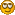TP you sure do come up with all kinds of things others should do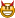Trouble Maker
« Last Edit: May 17, 2014, 12:56:13 PM by Ya-Nvr-No »Ya-Nvr-No•992